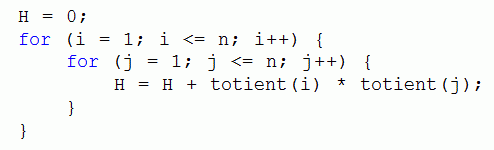Competitions

Totient Extreme

Given the value of n, you will have to find the value of H. The meaning of H is given in the following code:Totient or phi function, φ(n) is an arithmetic function that counts the number of positive integers less than or equal to n that are relatively prime to n. That is, if n is a positive integer, then φ(n) is the number of integers k in the range 1kn for which gcd(n, k) = 1.

Input

The first line contains the number of test cases t (0 < t106). It is followed by t lines each containing a number n (0 < n104).

Output

For each line produce one line that contains the value of H for the corresponding n.

Time limit 1 second
Memory limit 128 MiB
Input example #1
2
3
10
Output example #1
16
1024